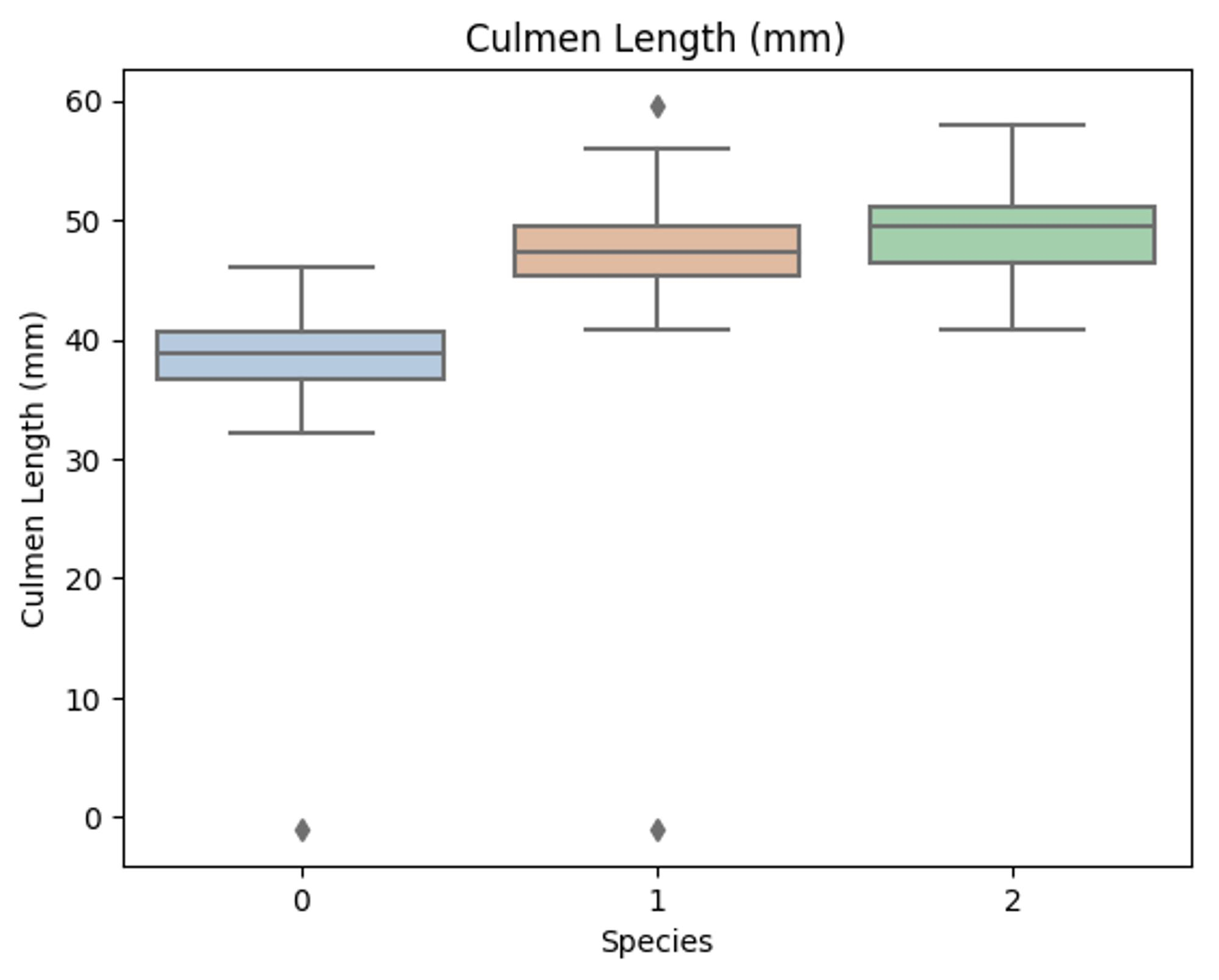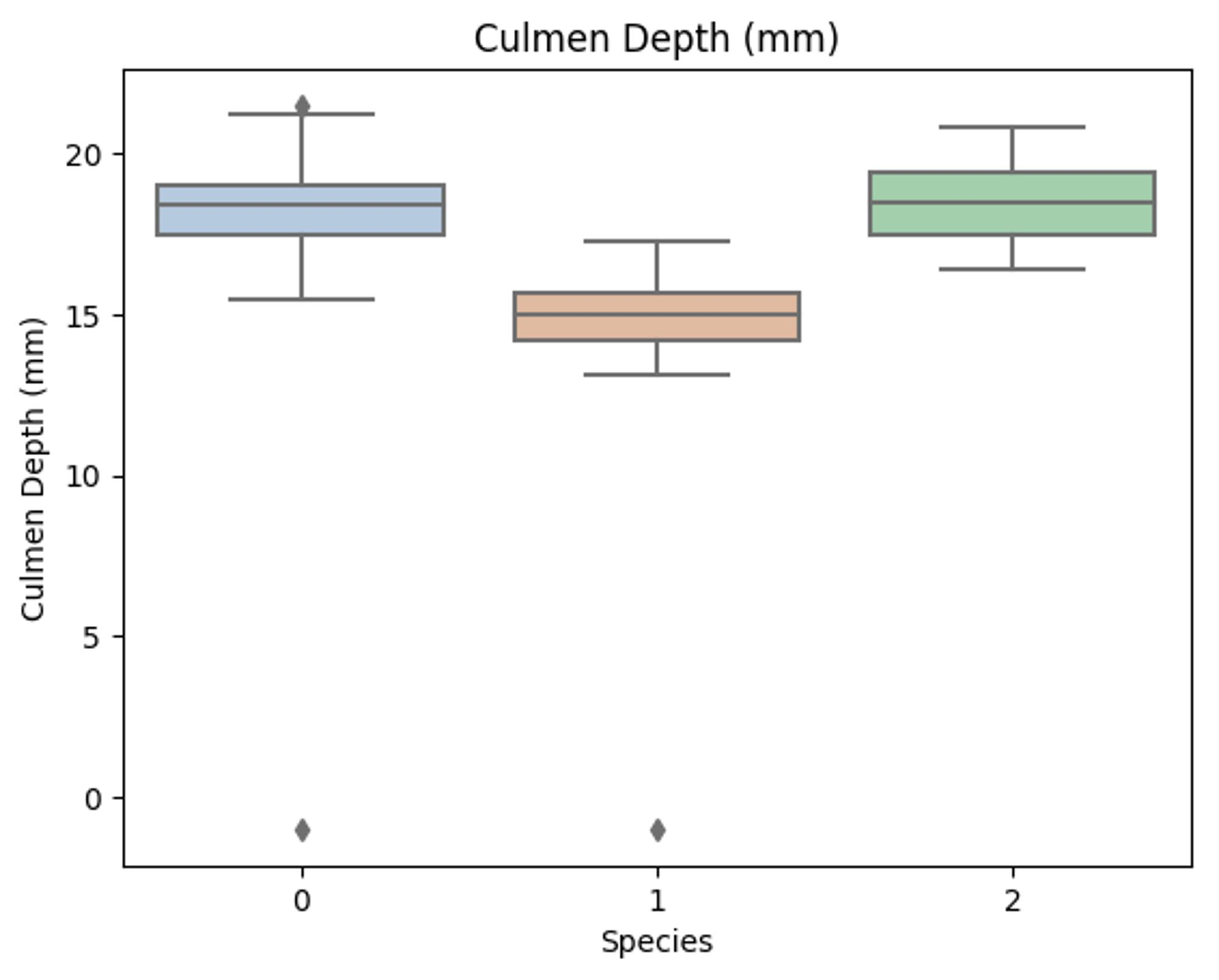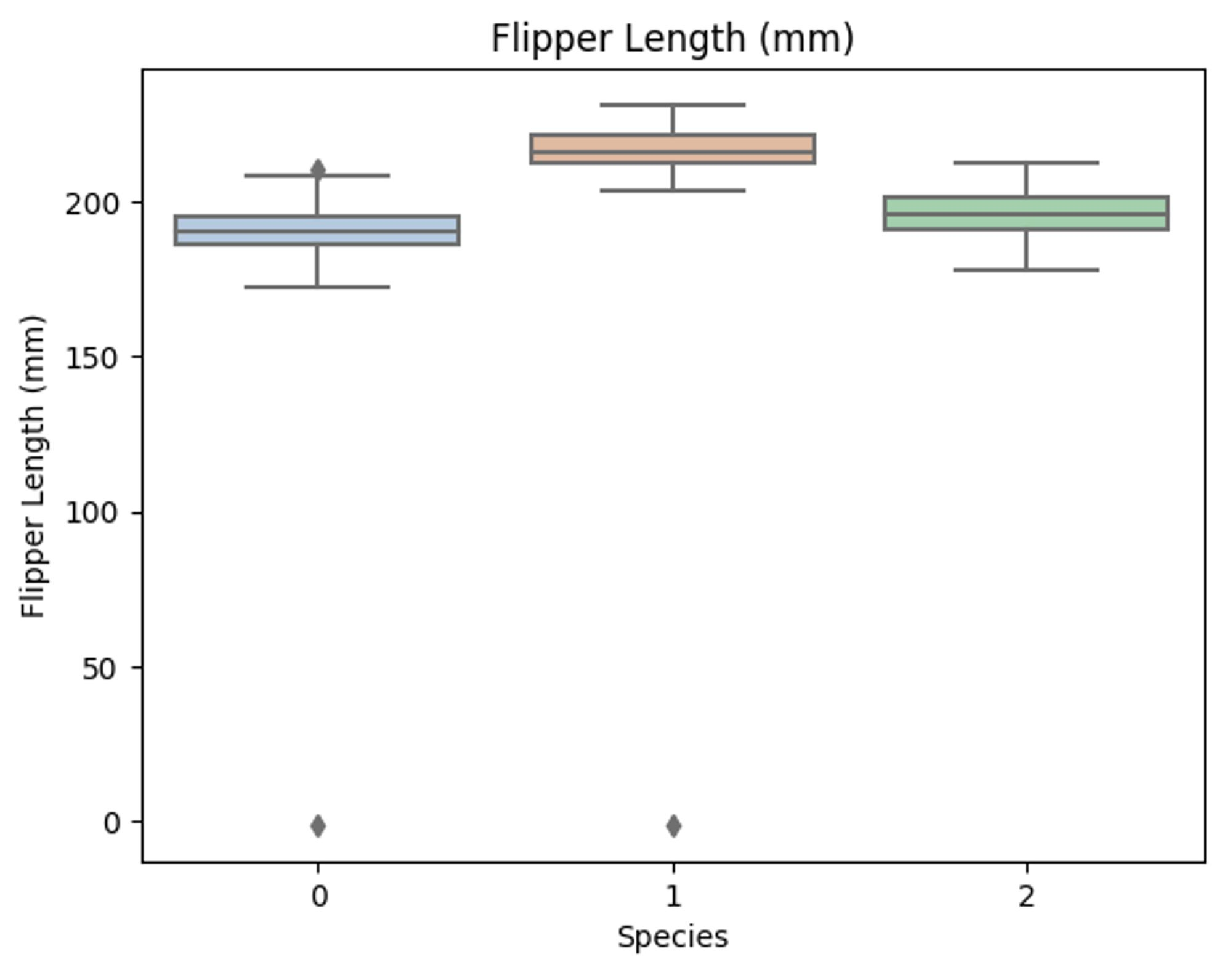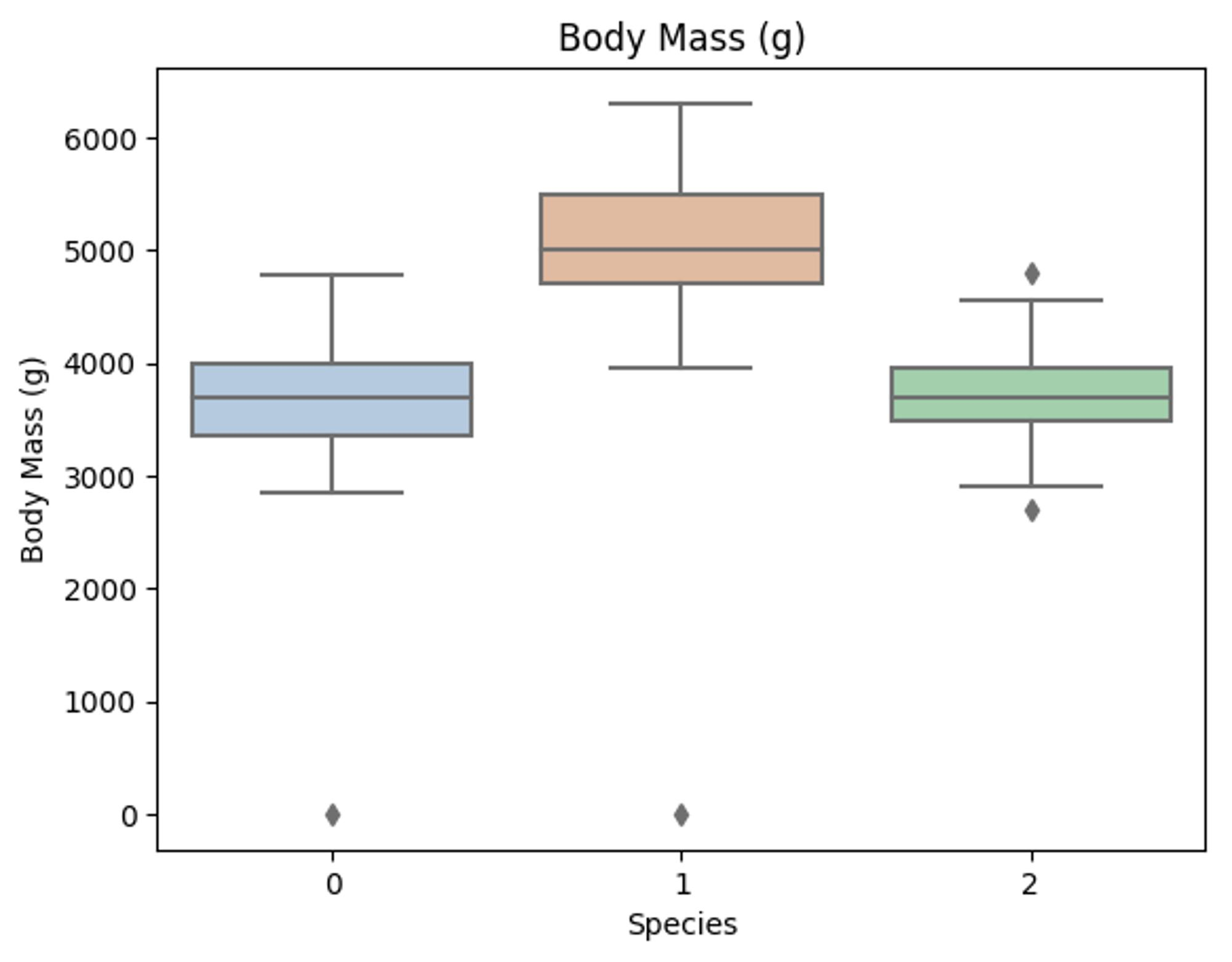V1

2023/03/25阅读：29主题：默认主题

# 1 逻决策树的介绍和应用

## 1.2 相关流程

• 了解 决策树 的理论知识
• 掌握 决策树 的 sklearn 函数调用并将其运用在企鹅数据集的预测中

Part1 Demo实践

• Step1:库函数导入
• Step2:模型训练
• Step3:数据和模型可视化
• Step4:模型预测

Part2 基于企鹅（penguins）数据集的决策树分类实践

• Step1:库函数导入
• Step2:数据读取/载入
• Step3:数据信息简单查看
• Step4:可视化描述
• Step5:利用 决策树模型 在二分类上 进行训练和预测
• Step6:利用 决策树模型 在三分类(多分类)上 进行训练和预测

# 3 算法实战

## 3.1Demo实践

Step1: 库函数导入

##  基础函数库import numpy as np ## 导入画图库import matplotlib.pyplot as pltimport seaborn as sns## 导入决策树模型函数from sklearn.tree import DecisionTreeClassifierfrom sklearn import tree

Step2: 训练模型

##Demo演示LogisticRegression分类## 构造数据集x_fearures = np.array([[-1, -2], [-2, -1], [-3, -2], [1, 3], [2, 1], [3, 2]])y_label = np.array([0, 1, 0, 1, 0, 1])## 调用决策树回归模型tree_clf = DecisionTreeClassifier()## 调用决策树模型拟合构造的数据集tree_clf = tree_clf.fit(x_fearures, y_label)

Step3: 数据和模型可视化（需要用到graphviz可视化库）

## 可视化构造的数据样本点plt.figure()plt.scatter(x_fearures[:,0],x_fearures[:,1], c=y_label, s=50, cmap='viridis')plt.title('Dataset')plt.show()
## 可视化决策树import graphvizdot_data = tree.export_graphviz(tree_clf, out_file=None)graph = graphviz.Source(dot_data)graph.render("pengunis")
'pengunis.pdf'


Step4:模型预测

## 创建新样本x_fearures_new1 = np.array([[0, -1]])x_fearures_new2 = np.array([[2, 1]])## 在训练集和测试集上分布利用训练好的模型进行预测y_label_new1_predict = tree_clf.predict(x_fearures_new1)y_label_new2_predict = tree_clf.predict(x_fearures_new2)print('The New point 1 predict class:\n',y_label_new1_predict)print('The New point 2 predict class:\n',y_label_new2_predict)
The New point 1 predict class:

The New point 2 predict class:



## 3.2 基于penguins_raw数据集的决策树实战

#下载需要用到的数据集!wget https://tianchi-media.oss-cn-beijing.aliyuncs.com/DSW/6tree/penguins_raw.csv
--2023-03-22 16:21:32--  https://tianchi-media.oss-cn-beijing.aliyuncs.com/DSW/6tree/penguins_raw.csv

penguins_raw.csv    100%[===================>]  51.85K  --.-KB/s    in 0.04s

2023-03-22 16:21:33 (1.23 MB/s) - 已保存 “penguins_raw.csv” [53098/53098])


Step1：函数库导入

##  基础函数库import numpy as np import pandas as pd## 绘图函数库import matplotlib.pyplot as pltimport seaborn as sns

species a factor denoting penguin species
island a factor denoting island in Palmer Archipelago, Antarctica
bill_length_mm a number denoting bill length
bill_depth_mm a number denoting bill depth
flipper_length_mm an integer denoting flipper length
body_mass_g an integer denoting body mass
sex a factor denoting penguin sex
year an integer denoting the study year

Step2：数据读取/载入

## 我们利用Pandas自带的read_csv函数读取并转化为DataFrame格式data = pd.read_csv('./penguins_raw.csv')
## 为了方便我们仅选取四个简单的特征，有兴趣的同学可以研究下其他特征的含义以及使用方法data = data[['Species','Culmen Length (mm)','Culmen Depth (mm)',            'Flipper Length (mm)','Body Mass (g)']]

Step3：数据信息简单查看

## 利用.info()查看数据的整体信息data.info()
<class 'pandas.core.frame.DataFrame'>
RangeIndex: 344 entries, 0 to 343
Data columns (total 5 columns):
#   Column               Non-Null Count  Dtype
---  ------               --------------  -----
0   Species              344 non-null    object
1   Culmen Length (mm)   342 non-null    float64
2   Culmen Depth (mm)    342 non-null    float64
3   Flipper Length (mm)  342 non-null    float64
4   Body Mass (g)        342 non-null    float64
dtypes: float64(4), object(1)
memory usage: 13.6+ KB

## 进行简单的数据查看，我们可以利用 .head() 头部.tail()尾部data.head()
Species Culmen Length (mm) Culmen Depth (mm) Flipper Length (mm) Body Mass (g)

data = data.fillna(-1)
data.tail()
Species Culmen Length (mm) Culmen Depth (mm) Flipper Length (mm) Body Mass (g)
339 Chinstrap penguin (Pygoscelis antarctica) 55.8 19.8 207.0 4000.0
340 Chinstrap penguin (Pygoscelis antarctica) 43.5 18.1 202.0 3400.0
341 Chinstrap penguin (Pygoscelis antarctica) 49.6 18.2 193.0 3775.0
342 Chinstrap penguin (Pygoscelis antarctica) 50.8 19.0 210.0 4100.0
343 Chinstrap penguin (Pygoscelis antarctica) 50.2 18.7 198.0 3775.0
## 其对应的类别标签为'Adelie Penguin', 'Gentoo penguin', 'Chinstrap penguin'三种不同企鹅的类别。data['Species'].unique()
array(['Adelie Penguin (Pygoscelis adeliae)',
'Gentoo penguin (Pygoscelis papua)',
'Chinstrap penguin (Pygoscelis antarctica)'], dtype=object)

## 利用value_counts函数查看每个类别数量pd.Series(data['Species']).value_counts()
Adelie Penguin (Pygoscelis adeliae)          152
Gentoo penguin (Pygoscelis papua)            124
Chinstrap penguin (Pygoscelis antarctica)     68
Name: Species, dtype: int64

## 对于特征进行一些统计描述data.describe()
Culmen Length (mm) Culmen Depth (mm) Flipper Length (mm) Body Mass (g)
count 344.000000 344.000000 344.000000 344.000000
mean 43.660756 17.045640 199.741279 4177.319767
std 6.428957 2.405614 20.806759 861.263227
min -1.000000 -1.000000 -1.000000 -1.000000
25% 39.200000 15.500000 190.000000 3550.000000
50% 44.250000 17.300000 197.000000 4025.000000
75% 48.500000 18.700000 213.000000 4750.000000
max 59.600000 21.500000 231.000000 6300.000000

Step4:可视化描述

## 特征与标签组合的散点可视化sns.pairplot(data=data, diag_kind='hist', hue= 'Species')plt.show()

'''为了方便我们将标签转化为数字       'Adelie Penguin (Pygoscelis adeliae)'        ------0       'Gentoo penguin (Pygoscelis papua)'          ------1       'Chinstrap penguin (Pygoscelis antarctica)   ------2 '''def trans(x):    if x == data['Species'].unique():        return 0    if x == data['Species'].unique():        return 1    if x == data['Species'].unique():        return 2data['Species'] = data['Species'].apply(trans)
for col in data.columns:    if col != 'Species':        sns.boxplot(x='Species', y=col, saturation=0.5, palette='pastel', data=data)        plt.title(col)        plt.show()# 选取其前三个特征绘制三维散点图from mpl_toolkits.mplot3d import Axes3Dfig = plt.figure(figsize=(10,8))ax = fig.add_subplot(111, projection='3d')data_class0 = data[data['Species']==0].valuesdata_class1 = data[data['Species']==1].valuesdata_class2 = data[data['Species']==2].values# 'setosa'(0), 'versicolor'(1), 'virginica'(2)ax.scatter(data_class0[:,0], data_class0[:,1], data_class0[:,2],label=data['Species'].unique())ax.scatter(data_class1[:,0], data_class1[:,1], data_class1[:,2],label=data['Species'].unique())ax.scatter(data_class2[:,0], data_class2[:,1], data_class2[:,2],label=data['Species'].unique())plt.legend()plt.show()

Step5:利用 决策树模型 在二分类上 进行训练和预测

## 为了正确评估模型性能，将数据划分为训练集和测试集，并在训练集上训练模型，在测试集上验证模型性能。from sklearn.model_selection import train_test_split## 选择其类别为0和1的样本 （不包括类别为2的样本）data_target_part = data[data['Species'].isin([0,1])][['Species']]data_features_part = data[data['Species'].isin([0,1])][['Culmen Length (mm)','Culmen Depth (mm)',            'Flipper Length (mm)','Body Mass (g)']]## 测试集大小为20%， 80%/20%分x_train, x_test, y_train, y_test = train_test_split(data_features_part, data_target_part, test_size = 0.2, random_state = 2020)
## 从sklearn中导入决策树模型from sklearn.tree import DecisionTreeClassifierfrom sklearn import tree## 定义 决策树模型 clf = DecisionTreeClassifier(criterion='entropy')# 在训练集上训练决策树模型clf.fit(x_train, y_train)
DecisionTreeClassifier(ccp_alpha=0.0, class_weight=None, criterion='entropy',
max_depth=None, max_features=None, max_leaf_nodes=None,
min_impurity_decrease=0.0, min_impurity_split=None,
min_samples_leaf=1, min_samples_split=2,
min_weight_fraction_leaf=0.0, presort='deprecated',
random_state=None, splitter='best')

## 可视化import graphvizdot_data = tree.export_graphviz(clf, out_file=None)graph = graphviz.Source(dot_data)graph.render("penguins")
'penguins.pdf'

## 在训练集和测试集上分布利用训练好的模型进行预测train_predict = clf.predict(x_train)test_predict = clf.predict(x_test)from sklearn import metrics## 利用accuracy（准确度）【预测正确的样本数目占总预测样本数目的比例】评估模型效果print('The accuracy of the Logistic Regression is:',metrics.accuracy_score(y_train,train_predict))print('The accuracy of the Logistic Regression is:',metrics.accuracy_score(y_test,test_predict))## 查看混淆矩阵 (预测值和真实值的各类情况统计矩阵)confusion_matrix_result = metrics.confusion_matrix(test_predict,y_test)print('The confusion matrix result:\n',confusion_matrix_result)# 利用热力图对于结果进行可视化plt.figure(figsize=(8, 6))sns.heatmap(confusion_matrix_result, annot=True, cmap='Blues')plt.xlabel('Predicted labels')plt.ylabel('True labels')plt.show()
The accuracy of the Logistic Regression is: 0.9954545454545455
The accuracy of the Logistic Regression is: 1.0
The confusion matrix result:
[[31  0]
[ 0 25]]


Step6:利用 决策树模型 在三分类(多分类)上 进行训练和预测

## 测试集大小为20%， 80%/20%分x_train, x_test, y_train, y_test = train_test_split(data[['Culmen Length (mm)','Culmen Depth (mm)',            'Flipper Length (mm)','Body Mass (g)']], data[['Species']], test_size = 0.2, random_state = 2020)## 定义 决策树模型 clf = DecisionTreeClassifier()# 在训练集上训练决策树模型clf.fit(x_train, y_train)
DecisionTreeClassifier(ccp_alpha=0.0, class_weight=None, criterion='gini',
max_depth=None, max_features=None, max_leaf_nodes=None,
min_impurity_decrease=0.0, min_impurity_split=None,
min_samples_leaf=1, min_samples_split=2,
min_weight_fraction_leaf=0.0, presort='deprecated',
random_state=None, splitter='best')

## 在训练集和测试集上分布利用训练好的模型进行预测train_predict = clf.predict(x_train)test_predict = clf.predict(x_test)## 由于决策树模型是概率预测模型（前文介绍的 p = p(y=1|x,\theta)）,所有我们可以利用 predict_proba 函数预测其概率train_predict_proba = clf.predict_proba(x_train)test_predict_proba = clf.predict_proba(x_test)print('The test predict Probability of each class:\n',test_predict_proba)## 其中第一列代表预测为0类的概率，第二列代表预测为1类的概率，第三列代表预测为2类的概率。## 利用accuracy（准确度）【预测正确的样本数目占总预测样本数目的比例】评估模型效果print('The accuracy of the Logistic Regression is:',metrics.accuracy_score(y_train,train_predict))print('The accuracy of the Logistic Regression is:',metrics.accuracy_score(y_test,test_predict))
The test predict Probability of each class:
[[0. 0. 1.]
[0. 1. 0.]
[0. 1. 0.]
[1. 0. 0.]
[1. 0. 0.]
[0. 0. 1.]
[0. 0. 1.]
[1. 0. 0.]
[0. 1. 0.]
[1. 0. 0.]
[0. 1. 0.]
[0. 1. 0.]
[1. 0. 0.]
[0. 1. 0.]
[0. 1. 0.]
[0. 1. 0.]
[1. 0. 0.]
[0. 1. 0.]
[1. 0. 0.]
[1. 0. 0.]
[0. 0. 1.]
[1. 0. 0.]
[0. 0. 1.]
[1. 0. 0.]
[1. 0. 0.]
[1. 0. 0.]
[0. 1. 0.]
[1. 0. 0.]
[0. 1. 0.]
[1. 0. 0.]
[1. 0. 0.]
[0. 0. 1.]
[0. 0. 1.]
[0. 1. 0.]
[1. 0. 0.]
[0. 1. 0.]
[0. 1. 0.]
[1. 0. 0.]
[1. 0. 0.]
[0. 1. 0.]
[0. 0. 1.]
[1. 0. 0.]
[0. 1. 0.]
[1. 0. 0.]
[1. 0. 0.]
[0. 0. 1.]
[0. 0. 1.]
[1. 0. 0.]
[1. 0. 0.]
[0. 1. 0.]
[1. 0. 0.]
[1. 0. 0.]
[0. 1. 0.]
[0. 1. 0.]
[0. 0. 1.]
[0. 0. 1.]
[0. 1. 0.]
[1. 0. 0.]
[1. 0. 0.]
[1. 0. 0.]
[0. 1. 0.]
[0. 1. 0.]
[0. 0. 1.]
[0. 0. 1.]
[1. 0. 0.]
[0. 1. 0.]
[0. 0. 1.]
[1. 0. 0.]
[1. 0. 0.]]
The accuracy of the Logistic Regression is: 0.9963636363636363
The accuracy of the Logistic Regression is: 0.9565217391304348

## 查看混淆矩阵confusion_matrix_result = metrics.confusion_matrix(test_predict,y_test)print('The confusion matrix result:\n',confusion_matrix_result)# 利用热力图对于结果进行可视化plt.figure(figsize=(8, 6))sns.heatmap(confusion_matrix_result, annot=True, cmap='Blues')plt.xlabel('Predicted labels')plt.ylabel('True labels')plt.show()
The confusion matrix result:
[[30  1  0]
[ 0 23  0]
[ 2  0 13]]


## 3.3 重要知识点

### 3.3.1 决策树构建的伪代码

1. 生成节点node
2. 中样本全书属于同一类别 :
3. ----将node标记为 类叶节点；
4. = 空集 OR D中样本在 上的取值相同 :
5. ----将node标记为叶节点，其类别标记为 中样本数最多的类；
6. 中选择最优划分属性 ;
7. 的每一个值 :
8. ----为node生成一个分支，令 表示 中在 上取值为 的样本子集；
9. ---- 为空 :
10. --------将分支节点标记为叶节点，其类别标记为 中样本最多的类;
11. ---- :
12. --------以 TreeGenerate( , { })为分支节点

### 3.3.3 重要参数

Criterion这个参数正是用来决定模型特征选择的计算方法的。sklearn提供了两种选择：

• 输入”entropy“，使用信息熵（Entropy）

• 输入”gini“，使用基尼系数（Gini Impurity）

random_state用来设置分枝中的随机模式的参数，默认None，在高维度时随机性会表现更明显。splitter也是用来控制决策树中的随机选项的，有两种输入值，输入”best"，决策树在分枝时虽然随机，但是还是会优先选择更重要的特征进行分枝（重要性可以通过属性feature_importances_查看），输入“random"，决策树在分枝时会更加随机，树会因为含有更多的不必要信息而更深更大，并因这些不必要信息而降低对训练集的拟合。

min_samples_leaf 限定，一个节点在分枝后的每个子节点都必须包含至少min_samples_leaf个训练样本，否则分枝就不会发生，或者，分枝会朝着满足每个子节点都包含min_samples_leaf个样本的方向去发生。一般搭配max_depth使用，在回归树中有神奇的效果，可以让模型变得更加平滑。这个参数的数量设置得太小会引起过拟合，设置得太大就会阻止模型学习数据。

# 4.总结

1. 具有很好的解释性，模型可以生成可以理解的规则。
2. 可以发现特征的重要程度。
3. 模型的计算复杂度较低。

1. 模型容易过拟合，需要采用减枝技术处理。
2. 不能很好利用连续型特征。
3. 预测能力有限，无法达到其他强监督模型效果。
4. 方差较高，数据分布的轻微改变很容易造成树结构完全不同。

• 对于机器学习这块规划为：基础入门机器学习算法--->简单项目实战--->数据建模比赛----->相关现实中应用场景问题解决。一条路线帮助大家学习，快速实战。
• 对于深度强化学习这块规划为：基础单智能算法教学（gym环境为主）---->主流多智能算法教学（gym环境为主）---->单智能多智能题实战（论文复现偏业务如：无人机优化调度、电力资源调度等项目应用）
• 自然语言处理相关规划：除了单点算法技术外，主要围绕知识图谱构建进行：信息抽取相关技术（含智能标注）--->知识融合---->知识推理---->图谱应用

1. 对于ML，希望你后续可以乱杀数学建模相关比赛（参加就获奖保底，top还是难的需要钻研）
2. 可以实际解决现实中一些优化调度问题，而非停留在gym环境下的一些游戏demo玩玩。（更深层次可能需要自己钻研了，难度还是很大的）
3. 掌握可知识图谱全流程构建其中各个重要环节算法，包含图数据库相关知识。V1